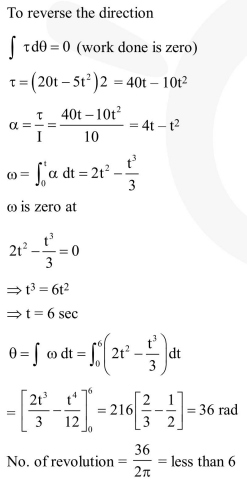Question:

A pulley of radius $2 \mathrm{~m}$ is rotated about its axis by a force $\mathrm{F}=\left(20 \mathrm{t}-5 \mathrm{t}^{2}\right)$ newton (where $\mathrm{t}$ is measured in seconds) applied tangentially. If the moment of inertia of the pulley about its axis of rotationis 10 $\mathrm{kg} \mathrm{m} \mathrm{m}^{2}$, the number of rotations made by the pulley before its direction of motion if reversed, is :-

1. more than 6 but less than 9

2. more than 9

3. less than 3

4. more than 3 but less than 6

Correct Option: , 4

Solution: